# Pedal curve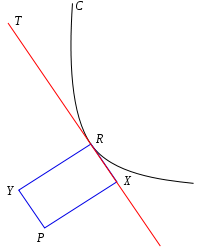Geometric construction of the pedal of C with respect to P

The pedal curve results from the orthogonal projection of a fixed point on the tangent lines of a given curve. More precisely, for a plane curve C and a given fixed pedal point P, the pedal curve of C is the locus of points X so that the line PX is perpendicular to a tangent T to the curve passing through the point X. Conversely, at any point R on the curve C, let T be the tangent line at that point R; then there is a unique point X on the tangent T which forms with the pedal point P a line perpendicular to the tangent T (for the special case when the fixed point P lies on the tangent T, the points X and P coincide) – the pedal curve is the set of such points X, called the foot of the perpendicular to the tangent T from the fixed point P, as the variable point R ranges over the curve C.

Complementing the pedal curve, there is a unique point Y on the line normal to C at R so that PY is perpendicular to the normal, so PXRY is a (possibly degenerate) rectangle. The locus of points Y is called the contrapedal curve.

The orthotomic of a curve is its pedal magnified by a factor of 2 so that the center of similarity is P. This is locus of the reflection of P through the tangent line T.

The pedal curve is the first in a series of curves C1, C2, C3, etc., where C1 is the pedal of C, C2 is the pedal of C1, and so on. In this scheme, C1 is known as the first positive pedal of C, C2 is the second positive pedal of C, and so on. Going the other direction, C is the first negative pedal of C1, the second negative pedal of C2, etc.

## Equations

### From the Cartesian equation

Take P to be the origin. For a curve given by the equation F(x, y)=0, if the equation of the tangent line at R=(x0, y0) is written in the formthen the vector (cos α, sin α) is parallel to the segment PX, and the length of PX, which is the distance from the tangent line to the origin, is p. So X is represented by the polar coordinates (p, α) and replacing (p, α) by (r, θ) produces a polar equation for the pedal curve.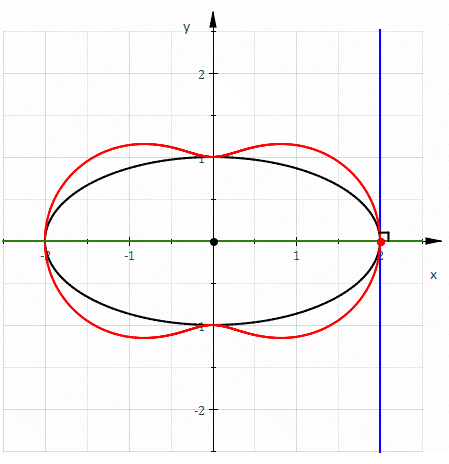Pedal curve (red) of an ellipse (black). Here a=2 and b=1 so the equation of the pedal curve is 4x2+y2=(x2+y2)2

For example, for the ellipsethe tangent line at R=(x0, y0) isand writing this in the form given above requires thatThe equation for the ellipse can be used to eliminate x0 and y0 givingand converting to (r, θ) givesas the polar equation for the pedal. This is easily converted to a Cartesian equation as### From the polar equation

For P the origin and C given in polar coordinates by r = f(θ). Let R=(r, θ) be a point on the curve and let X=(p, α) be the corresponding point on the pedal curve. Let ψ denote the angle between the tangent line and the radius vector, sometimes known as the polar tangential angle. It is given byThenandThese equations may be used to produce an equation in p and α which, when translated to r and θ gives a polar equation for the pedal curve.

For example, let the curve be the circle given by r = a cos θ. ThensoAlsoSo the polar equation of the pedal is### From the pedal equation

The pedal equations of a curve and its pedal are closely related. If P is taken as the pedal point and the origin then it can be shown that the angle ψ between the curve and the radius vector at a point R is equal to the corresponding angle for the pedal curve at the point X. If p is the length of the perpendicular drawn from P to the tangent of the curve (i.e. PX) and q is the length of the corresponding perpendicular drawn from P to the tangent to the pedal, then by similar trianglesIt follows immediately that the if the pedal equation of the curve is f(p,r)=0 then the pedal equation for the pedal curve isFrom this all the positive and negative pedals can be computed easily if the pedal equation of the curve is known.

### From parametric equations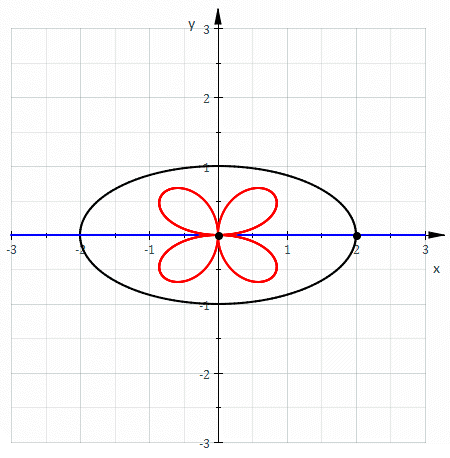Contrapedal of the same ellipse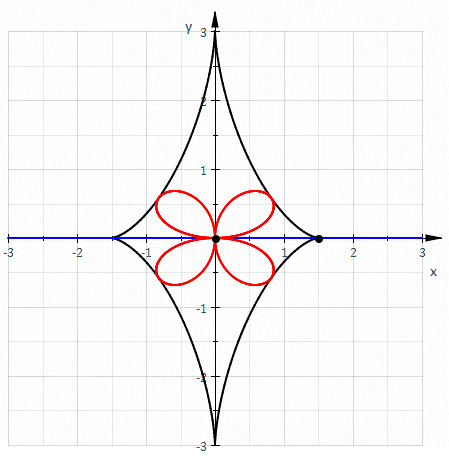Pedal of the evolute of the ellipse : same as the contrapedal of the original ellipse

Letbe the vector for R to P and write,

the tangential and normal components ofwith respect to the curve. Thenis the vector from R to X from which the position of X can be computed.

Specifically, if c is a parametrization of the curve thenparametrises the pedal curve (disregarding points where c' is zero or undefined).

For a parametrically defined curve, its pedal curve with pedal point (0;0) is defined asThe contrapedal curve is given by:With the same pedal point, the contrapedal curve is the pedal curve of the evolute of the given curve.

## Geometrical properties

Consider a right angle moving rigidly so that one leg remains on the point P and the other leg is tangent to the curve. Then the vertex of this angle is X and traces out the pedal curve. As the angle moves, its direction of motion at P is parallel to PX and its direction of motion at R is parallel to the tangent T = RX. Therefore, the instant center of rotation is the intersection of the line perpendicular to PX at P and perpendicular to RX at R, and this point is Y. If follows that the tangent to the pedal at X is perpendicular to XY.

Draw a circle with diameter PR, then it circumscribes rectangle PXRY and XY is another diameter. The circle and the pedal are both perpendicular to XY so they are tangent at X. Hence the pedal is the envelope of the circles with diameters PR where R lies on the curve.

The line YR is normal to the curve and the envelope of such normals is its evolute. Therefore, YR is tangent to the evolute and the point Y is the foot of the perpendicular from P to this tangent, in other words Y is on the pedal of the evolute. It follows that the contrapedal of a curve is the pedal of its evolute.

Let C′ be the curve obtained by shrinking C by a factor of 2 toward P. Then the point R′ corresponding to R is the center of the rectangle PXRY, and the tangent to C′ at R′ bisects this rectangle parallel to PY and XR. A ray of light starting from P and reflected by C′ at R' will then pass through Y. The reflected ray, when extended, is the line XY which is perpendicular to the pedal of C. The envelope of lines perpendicular to the pedal is then the envelope of reflected rays or the catacaustic of C′. This proves that the catacaustic of a curve is the evolute of its orthotomic.

As noted earlier, the circle with diameter PR is tangent to the pedal. The center of this circle is R′ which follows the curve C′. It follows that the envelope of circles through a fixed point and whose centers lie on a given curve is the orthotomic of the curve.

Let D′ be a curve congruent to C′ and let D′ roll without slipping, as in the definition of a roulette, on C′ so that D′ is always the reflection of C′ with respect to the line to which they are mutually tangent. Then when the curves touch at R′ the point corresponding to P on the moving plane is X, and so the roulette is the pedal curve. Equivalently, the orthotomic of a curve is the roulette of the curve on its mirror image.

### Example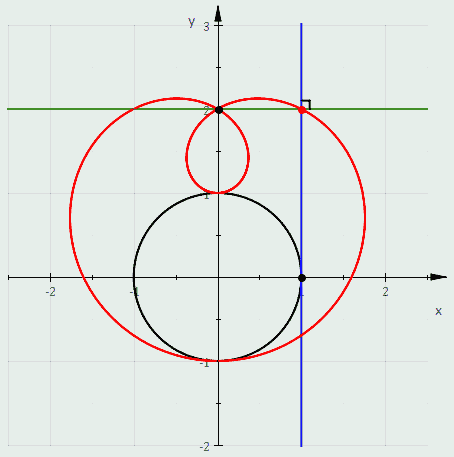Limaçon — pedal curve of a circle

When C is a circle the above discussion shows that the following definitions of a limaçon are equivalent:

• It is the pedal of a circle.
• It is the envelope of circles whose diameters have one endpoint on a fixed point and another endpoint which follow a circle.
• It is the envelope of circles through a fixed point whose centers follow a circle.
• It is the roulette formed by a circle rolling around a circle with the same radius.

We also have shown that the catacaustic of a circle is the evolute of a limaçon.

## Pedals of specific curves

Pedals of some specific curves are:

Curve Equation Pedal point Pedal curve
Circle Point on circumference Cardioid
Circle Any point Limaçon
Parabola Focus The tangent line at the vertex
Parabola Vertex Cissoid of Diocles
Central conic Focus Auxiliary circle
Central conicCenter(a hippopede)
Rectangular hyperbola Center Lemniscate of Bernoulli
Logarithmic spiral Pole Logarithmic spiral
Sinusoidal spiralPole(another Sinusoidal spiral)

Notes

1. Edwards p. 165
2. Edwards p. 164
3. Follows Edwards p. 164 with m=1
4. Edwards p. 164-5
5. Follows Edwards p. 165 with m=1
6. Williamson p. 228
7. Edwards p. 167

SourcesWikimedia Commons has media related to Pedal curves.